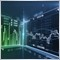# New metatrader 4 compatible indicators - page 327160786

An example of an "as simple as it gets", non-repainting two color multi time frame average that is coded to the last letter using the new metatrader 4 builds coding possibilities (and conventions)

```//------------------------------------------------------------------
//------------------------------------------------------------------
#property indicator_chart_window
#property indicator_buffers 3
#property indicator_color1 clrLimeGreen
#property indicator_color2 clrSandyBrown
#property indicator_color3 clrSandyBrown
#property indicator_width1 3
#property indicator_width2 3
#property indicator_width3 3
#property strict

extern ENUM_TIMEFRAMES    TimeFrame = PERIOD_CURRENT; // Time frame to use
extern int                MAPeriod  = 12;             // Average period
extern ENUM_APPLIED_PRICE MAPrice   = PRICE_CLOSE;    // Price
extern ENUM_MA_METHOD     MAMethod  = MODE_LWMA;      // Average method
extern int                MAShift   = 0;              // Shift
extern bool               Interpolate = true;         // Interpolate in multi time frame mode

//
//
//
//
//

string indicatorFileName;
bool   returnBars;

//------------------------------------------------------------------
//
//------------------------------------------------------------------
//
//
//
//
//

int OnInit()
{
IndicatorBuffers(4);
SetIndexBuffer(0,ma);
SetIndexBuffer(3,slope);
indicatorFileName = WindowExpertName();
returnBars        = TimeFrame==-99;
TimeFrame         = MathMax(TimeFrame,_Period);
for (int i=0; i<3; i++) SetIndexShift(i,MAShift*TimeFrame/_Period);
return(INIT_SUCCEEDED);
}
void OnDeinit(const int reason) { }

//------------------------------------------------------------------
//
//------------------------------------------------------------------
//
//
//
//
//

int OnCalculate(const int rates_total,
const int prev_calculated,
const datetime &time[],
const double   &open[],
const double   &high[],
const double   &low[],
const double   &close[],
const long     &tick_volume[],
const long     &volume[],
{
int counted_bars = prev_calculated;
if(counted_bars < 0) return(-1);
if(counted_bars > 0) counted_bars--;
int limit=MathMin(rates_total-counted_bars,rates_total-1);
if (returnBars) { ma = MathMin(limit+1,rates_total-1); return(rates_total); }
if (TimeFrame!=_Period) limit = (int)MathMax(limit,MathMin(rates_total-1,iCustom(NULL,TimeFrame,indicatorFileName,-99,0,0)*TimeFrame/_Period));

//
//
//
//
//

for(int i = limit; i >= 0; i--)
{
int y = iBarShift(NULL,TimeFrame,time[i]);
ma[i]    = iMA(NULL,TimeFrame,MAPeriod,0,MAMethod,MAPrice,y);
slope[i] = (i<rates_total-1) ? (ma[i]>ma[i+1]) ? 1 : (ma[i]<ma[i+1]) ? -1 : slope[i+1] : 0;

if (!Interpolate || (i>0 && y==iBarShift(NULL,TimeFrame,time[i-1]))) continue;
int n,k; datetime ctime = iTime(NULL,TimeFrame,y);
for(n = 1; i+n < rates_total && time[i+n] >=ctime; n++) continue;
for(k = 1; i+n < rates_total && i+k < rates_total && k<n; k++)  ma[i+k] = ma[i] + (ma[i+n]-ma[i])*k/n;
}
return(rates_total);
}

//-------------------------------------------------------------------
//
//-------------------------------------------------------------------
//
//
//
//
//

void CleanPoint(int i,double& first[],double& second[])
{
if (i>=Bars-3) return;
if ((second[i]  != EMPTY_VALUE) && (second[i+1] != EMPTY_VALUE))
second[i+1] = EMPTY_VALUE;
else
if ((first[i] != EMPTY_VALUE) && (first[i+1] != EMPTY_VALUE) && (first[i+2] == EMPTY_VALUE))
first[i+1] = EMPTY_VALUE;
}

void PlotPoint(int i,double& first[],double& second[],double& from[])
{
if (i>=Bars-2) return;
if (first[i+1] == EMPTY_VALUE)
if (first[i+2] == EMPTY_VALUE)
{ first[i]  = from[i];  first[i+1]  = from[i+1]; second[i] = EMPTY_VALUE; }
else  { second[i] =  from[i]; second[i+1] = from[i+1]; first[i]  = EMPTY_VALUE; }
else     { first[i]  = from[i];                           second[i] = EMPTY_VALUE; }
}```

PS: do not confuse the code with the metatrader 5 code. Even though they look very similar, due to fundamental differences between metatrader 4 and 5, this code can not be just compiled using metatrader 5 and work - some serious coding changes would need to be done before that could happen. So, there is no "easy way out" for mt4 to mt5 conversion11

And this one
Windows property left, which is now added ..
Files:53

Dear mladen does it need NMC not attaching to chart
Files:160786

forexislife:
Dear mladen does it need NMC not attaching to chart
No. All it needs is the zigzag indicator to run53

No. All it needs is the zigzag indicator to run
so no use because it repaints195

Dear  mladen does it need any NMC , not updating please check out160786

shiva12:
Dear  mladen does it need any NMC , not updating please check out

shiva12

I would need the original (non-decompiled) source code tio check what is wrong with it195

Hi all does any one have source file for this indicator

Files:160786

shiva12:

Hi all does any one have source file for this indicator

That is the old version of these :

Files:323